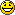Rotary Encoder - SPARTAN 3E - VHDLRotary Encoder - SPARTAN 3E - VHDL

this is a small program wrote in VHDL to work with the Rotary Encoder:

----------------------------------------------------------------------------------
-- Company: CNESTEN
-- Abed OUBARI
-- Create Date: 10:52:14 10/06/2011
-- Design Name:
-- Module Name: rot_s - Behavioral
-- Project Name:
-- Rotary Encoder
-- Spartan 3E
-- VHDL
--
--
--
-- year: 2011
----------------------------------------------------------------------------------
library IEEE;
use IEEE.STD_LOGIC_1164.ALL;

-- Uncomment the following library declaration if using
-- arithmetic functions with Signed or Unsigned values
--use IEEE.NUMERIC_STD.ALL;

-- Uncomment the following library declaration if instantiating
-- any Xilinx primitives in this code.
--library UNISIM;
--use UNISIM.VComponents.all;

entity rot_s is
Port ( clk : in STD_LOGIC;
rot_a : in STD_LOGIC;
rot_b : in STD_LOGIC;
rot_center : in STD_LOGIC;
led : out STD_LOGIC_VECTOR (7 downto 0));
end rot_s;

architecture Behavioral of rot_s is

signal rotary_a_in: std_logic;
signal rotary_b_in: std_logic;
signal rotary_q1: std_logic;
signal rotary_q2: std_logic;
signal rotary_in: std_logic_vector(1 downto 0);
signal rotary_event: std_logic;
signal rotary_left:std_logic;
signal delay_rotary_q1:std_logic;
signal center_flag:std_logic;

begin

rotary_a_in <= rot_a;
rotary_b_in <= rot_b;

rotary_filter: process(clk)
begin
if clk'event and clk='1' then
rotary_in <= rotary_b_in & rotary_a_in;

case rotary_in is
when "00" => rotary_q1 <= '0';
rotary_q2 <= rotary_q2;
when "01" => rotary_q1 <= rotary_q1;
rotary_q2 <= '0';
when "10" => rotary_q1 <= rotary_q1;
rotary_q2 <= '1';
when "11" => rotary_q1 <= '1';
rotary_q2 <= rotary_q2;
when others => rotary_q1 <= rotary_q1;
rotary_q2 <= rotary_q2;
end case;
end if;
end process rotary_filter;

direction: process(clk)
begin
if clk'event and clk='1' then

delay_rotary_q1 <= rotary_q1;
if rotary_q1='1' and delay_rotary_q1='0' then
rotary_event <= '1';
rotary_left <= rotary_q2;
else
rotary_event <= '0';
rotary_left <= rotary_left;
end if;
end if;
end process direction;

led_switch: process(clk,rotary_event,rotary_left)

variable i : integer;
variable index : integer;
begin
if clk'event and clk='1' then
if rotary_event='1' and rotary_left='0' then --left
led <="00000000";
i:=i+1;
index :=i mod 8;
led(index)<='1';
end if;
if rotary_event='1' and rotary_left='1' then --right
led <="00000000";
if i=0 then
i:=8;
end if;
i:=i-1;
index :=i mod 8;
led(index)<='1';
end if;
end if;
end process led_switch;

process(rot_center)
begin
if (rot_center='1') then
center_flag<='1';
elsif (rot_center='0') then
center_flag<='0';
end if;
end process;
end Behavioral;

Let me know if it works fne!
Have funabed.oubari

Posts : 77
Join date : 2010-02-25
Age : 40
Location : MoroccoRe: Rotary Encoder - SPARTAN 3E - VHDL

Hi.. let me introduce my self..

can u explain it to me about your code,, but not all.. i still can undrstand some part of ur code..

rotary_a_in <= rot_a;
rotary_b_in <= rot_b;

rotary_filter: process(clk)
begin
if clk'event and clk='1' then
rotary_in <= rotary_b_in & rotary_a_in;

case rotary_in is
when "00" => rotary_q1 <= '0';
rotary_q2 <= rotary_q2;
when "01" => rotary_q1 <= rotary_q1;
rotary_q2 <= '0';
when "10" => rotary_q1 <= rotary_q1;
rotary_q2 <= '1';
when "11" => rotary_q1 <= '1';
rotary_q2 <= rotary_q2;
when others => rotary_q1 <= rotary_q1;
rotary_q2 <= rotary_q2;
end case;
end if;
end process rotary_filter;

1. what the meaning of
Code:
`when "00" => rotary_q1 <= '0';`
?
2. what the fuction of rotary_q1 and rotary_q2?

im very thank you if u reply my message and want explain it...

Regards,

viduka

Posts : 2
Join date : 2012-02-17Hi!

To understand the code below you need a PDF document that explain everything, I can send it to you. please contact me :

abed.oubari@gmail.com

(rotary-q1 and rotary-q2 are two counters)abed.oubari

Posts : 77
Join date : 2010-02-25
Age : 40
Location : MoroccoRe: Rotary Encoder - SPARTAN 3E - VHDL